Superconductivity

This free course is available to start right now. Review the full course description and key learning outcomes and create an account and enrol if you want a free statement of participation.

Free course

3.4 Penetration depth

The characteristic length, λ, associated with the decay of the magnetic field at the surface of a superconductor is known as the penetration depth, and it depends on the number density n﻿s of superconducting electrons.

We can estimate a value for λ by assuming that all of the free electrons are superconducting. If we set n﻿s = 10﻿29 m﻿−﻿3, a typical free electron density in a metal, then we find that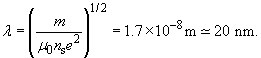The small size of λ indicates that the magnetic field is effectively excluded from the interior of macroscopic specimens of superconductors, in agreement with the experimentally observed Meissner effect.

The small scale of the field penetration means that carefully-designed experiments are needed to measure the value of λ. Many experiments have been done with samples that have a large surface to volume ratio to make the penetration effect of the field appreciable. Thin films, thin wires and colloidal particles of superconductors have all been used for this purpose. But it is also possible to use large specimens if the measurement is sensitive to the amount of magnetic flux passing through the superconductor's surface, and not to the ratio of flux excluded by the superconductor to flux through the normal material, which is close to unity.

In a classic experiment performed in the 1950s, Schawlow and Devlin measured the self-inductance of a solenoid within which they inserted a long single-crystal cylinder of superconducting tin, 7.4 mm in diameter. They minimised the space between the coil and the tin cylinder, and since no magnetic flux passed through the bulk of the superconductor, the flux was essentially restricted to a thin cylindrical shell of thickness λ at the surface of the cylinder. The inductance of the solenoid was therefore determined mainly by the magnitude of the penetration depth. To measure the inductance, a capacitor was connected in parallel with the solenoid, and the natural angular frequency,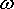n = 1/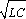, of the LC circuit was measured. The precision of the frequency measurement was about one part in 10﻿6, which corresponded to a precision of 0.4 nm in the value of the penetration depth. The result that they obtained for the penetration depth of tin for temperatures much lower than the critical temperature was 52 nm.

The number density of superconducting electrons depends on temperature, so the penetration depth is temperature dependent. For T ≪ T﻿c, all of the free electrons are superconducting, but the number density falls steadily with increasing temperature until it reaches zero at the critical temperature. Since λns-1/2 according to the London model, the penetration depth increases as the temperature approaches the critical temperature, becoming effectively infinite – corresponding to a uniform field in the material – at and above the critical temperature. Figure 16 shows this temperature dependence for tin, which is well represented by the expression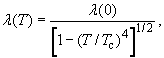where λ(0) is the value of the penetration depth at T = 0 K.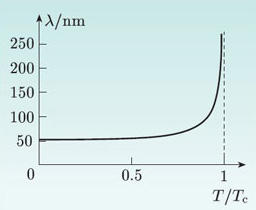Figure 16 The penetration depth λ as a function of temperature for tin.

SMT359_1